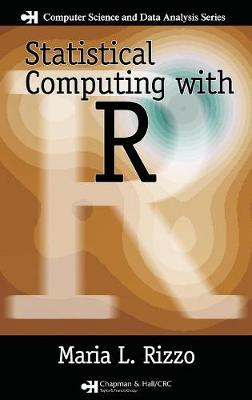•# Statistical Computing with R - Chapman & Hall/CRC: The R Series (Hardback)

(author)
£53.99
Hardback 416 Pages / Published: 15/11/2007
• Not available

This product is currently unavailable.

Computational statistics and statistical computing are two areas that employ computational, graphical, and numerical approaches to solve statistical problems, making the versatile R language an ideal computing environment for these fields. One of the first books on these topics to feature R, Statistical Computing with R covers the traditional core material of computational statistics, with an emphasis on using the R language via an examples-based approach. Suitable for an introductory course in computational statistics or for self-study, it includes R code for all examples and R notes to help explain the R programming concepts.

After an overview of computational statistics and an introduction to the R computing environment, the book reviews some basic concepts in probability and classical statistical inference. Each subsequent chapter explores a specific topic in computational statistics. These chapters cover the simulation of random variables from probability distributions, the visualization of multivariate data, Monte Carlo integration and variance reduction methods, Monte Carlo methods in inference, bootstrap and jackknife, permutation tests, Markov chain Monte Carlo (MCMC) methods, and density estimation. The final chapter presents a selection of examples that illustrate the application of numerical methods using R functions.

Focusing on implementation rather than theory, this text serves as a balanced, accessible introduction to computational statistics and statistical computing.

Publisher: Taylor & Francis Inc
ISBN: 9781584885450
Number of pages: 416
Weight: 726 g
Dimensions: 235 x 156 x 28 mm